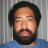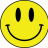S

#### stocksniper

##### Member
Code:
``````## Scalper's Helper w/ Squeeze,  by Linus, Version = 2013-11-12.2
#hint: The Count plot adds the sum of price momentum comparisons with a counter of concurrent bars with no signaled direction change.  The Sig plot is a signal line colored to show if in or out of the squeeze.  (Lighter color is out of squeeze, by default.) \n\n Possible uses: \n\n If current Count is greater than previous Count of a different direction, and if not in the squeeze, look for possible breakout/breakdown or extension. \n\n If current Count is below Sig and previous Count was way above Sig, look for possible price direction change. \n\n Notes: \n\n Direction changes occur when the PPS signal changes and all the filters for that direction are either off or valid.  The Count plot is colored to show the current direction. (Green for up and Red for down, by default.)

declare lower;
input paintBars = No;    # hint paintBars: Yes to color price bars.
input diFilter = Yes;    #hint  diFilter: No to turn off DI filter.
input avgFilter = Yes;   #hint avgFilter: No to turn off mov. avg. filter.
input prFilter = Yes;    #hint prFilter: No to turn off price filter.
input price = close;     #hint price:  price fundamental.
input prMom = 1;         #hint prMom: offset for price (mom)entum.
input diLength = 13;     #hint diLength: For DI+, DI- calc, and mom sum.
input signal = 13;       #hint signal: Horiz. position of the Sig line.

#############################################
# Keltner Channel and Bollinger Band Squeeze:
input avgType = AverageType.SIMPLE; # Mov. avg type.
input avgLength = 20;    # length for band and channel mov. avg.
input numDev = 2.0;      # number of std devs of band.
input factor = 1.5;      # factor for offsetting channel.
def MA = MovingAverage(avgType, price, avgLength);

# inSqueeze is true if the upper band is inside the upper channel:
def inSqueeze = (MA + numDev * StDev(price, avgLength)) < (MA + (factor * Average(TrueRange(high,  close,  low),  avgLength)));

#
#############################################
def hiDiff = high - high;
def loDiff = low - low;
def ATR = WildersAverage(TrueRange(high, close, low), diLength);
# diDif is ("DI+" - "DI-")
def diDif = (100 * WildersAverage(if hiDiff > loDiff and hiDiff > 0 then hiDiff else 0, diLength) / ATR) - (100 * WildersAverage(if loDiff > hiDiff and loDiff > 0 then loDiff else 0, diLength) / ATR);

def ppsDir = CompoundValue(1, if !IsNaN(PPS().BuySignal) then 1 else if !IsNaN(PPS().SellSignal) then -1 else ppsDir, 0);

def diUp = !diFilter or (diFilter and diDif > 0 and diDif > diDif);
def diDn = !diFilter or (diFilter and diDif < 0 and diDif < diDif);
def avgUp = !avgFilter or (avgFilter and price > MA);
def avgDn = !avgFilter or (avgFilter and price < MA);

# Price momentum sums:
def sumUp = if prFilter then Sum(price > price[prMom], diLength) else 0;
def sumDn = if prFilter then Sum(price < price[prMom], diLength) else 0;
# prLbl=Off: Hide price momentum label.
# prLbl=All: Show prMom, sumUp and sumDn values.
# prLbl=UD:  Show only sumUp and sumDn values.

input prLbl = {default Off, All, UD};

AddLabel(prLbl != prLbl.Off, (if prLbl == prLbl.All then "prMom=" + prMom + " :: " else "") + "U=" + sumUp + " :: D=" + sumDn, if sumUp > sumDn then Color.GREEN else if sumUp < sumDn then Color.RED else Color.GRAY);

# dir is 1 if last PPS signal was up, and up filters are valid or turned off;  dir is -1 if last PPS signal was down, and down filters are valid or turned off.

def dir = CompoundValue(1, if ppsDir > 0 and avgUp and diUp then 1 else if ppsDir < 0 and avgDn and diDn then -1 else dir, 0);

# Count the number of times dir is in one direction.
def dirCnt = compoundValue(1, if dir crosses 0 then 1 else dirCnt + 1, 0);

# If dir is up (> 0) then add to dirCnt the sum of price bars moving up, else add to dir the sum of price bars moving down.

plot Count = if !IsNaN(close) then
if dir > 0 then dirCnt + sumUp else dirCnt + sumDn
else Double.NaN;

# Sig is colored differently when in or out of squeeze.
plot Sig = if !IsNaN(close) then signal else Double.NaN;
Sig.SetPaintingStrategy(PaintingStrategy.POINTS);
Sig.AssignValueColor(if inSqueeze then Color.DARK_GRAY else Color.WHITE);
Sig.SetDefaultColor(Color.GRAY);
Count.SetPaintingStrategy(PaintingStrategy.LINE_VS_POINTS);
Count.AssignValueColor(if dir > 0 then if Count > Sig then Color.GREEN else Color.DARK_GREEN else if Count > Sig then Color.RED else Color.DARK_RED);

AssignPriceColor(if !paintBars then Color.CURRENT else if dir > 0 then if Count > Sig then Color.GREEN  else Color.GREEN else if dir < 0 then if Count > Sig then Color.RED else Color.DARK_RED else Color.GRAY);``````

Last edited by a moderator:
S

#### stocksniper

##### Member
How can I create this into a scan?

T

#### tomsk

##### Well-known member
VIP
I took a look at the study you posted above, it has 2 plot statements

(1) Count plot - adds the sum of price momentum comparisons with a counter of concurrent bars with no signaled direction change.
(2) Sig plot - a signal line colored to show if in or out of the squeeze.

This study has no buy/sell signals
What exactly are you attempting to scan for?

S

#### stocksniper

##### Member
When the count turns green, its a buy signal I dont use the sig plot only the count plot and it has giving me some nice entries. So I would like to scan when count plot turns green for the first time.

T

#### tomsk

##### Well-known member
VIP
I looked through the code, and within it there are multiple dependencies on another TOS study called "PPS" for which the source code is locked and not available for the general public. Hence converting this into a scan would be problematic. Additionally the structure of the underlying code as currently written would be complex for the scan engine

Last edited:#### Townsend

##### Active member
VIP
To the best of my knowledge TOS does not allow user selected .wav or .mp3 files. Rather... the only sounds you can play are the system sounds: Bell, Ding, Ring, or Chimes. Adding a sound to your code is simple using the Alert command.

Code:
``Alert (IDataHolder condition,  String text,  int alert type,  String sound);``

T

#### tomsk

##### Well-known member
VIP
@stocksniper Good news - it turns out that when I was looking into your request on Friday, TOS was doing some maintenance work which impacted the overall performance of the scanner. I restructured some of the code logic, cleaned up the code and successfully converted your study into a scan. Since the PPS code was locked and not available, I had several versions of the scan going using a replica PPS study. Since then I was able to use the TOS PPS, but there seems to be some lag in getting scan results.

Note that there are two plot statements in the scan. I have configured this for the bullish case.
If you'd like to use the bearish case scan, just comment out the bullish scan and uncomment the bearish scan. All this is listed at the end of the file

Here then is the converted scan

Code:
``````# Scalper's Helper Scan
# tomsk
# 12.1.2019
#

# V2.1 - 11.30.2019 - tomsk - Converted to scan, and using replica PPS script() function
# V2.2 - 12.01.2019 - tomsk - Restructured code logic, and reverted back to standard TOS PPS

## Scalper's Helper w/ Squeeze,  by Linus, Version = 2013-11-12.2
#hint: The Count plot adds the sum of price momentum comparisons with a counter of concurrent bars with no signaled direction change.  The Sig plot is a signal line colored to show if in or out of the squeeze.  (Lighter color is out of squeeze, by default.) \n\n Possible uses: \n\n If current Count is greater than previous Count of a different direction, and if not in the squeeze, look for possible breakout/breakdown or extension. \n\n If current Count is below Sig and previous Count was way above Sig, look for possible price direction change. \n\n Notes: \n\n Direction changes occur when the PPS signal changes and all the filters for that direction are either off or valid.  The Count plot is colored to show the current direction. (Green for up and Red for down, by default.)

input diFilter = Yes;    # No to turn off DI filter.
input avgFilter = Yes;   # No to turn off mov. avg. filter.
input prFilter = Yes;    # No to turn off price filter.
input price = close;
input prMom = 1;         # offset for price momentum.
input diLength = 13;     # For DI+, DI- calc, and mom sum. Default 14
input signal = 13;       # Horiz. position of the Sig line.

# Keltner Channel / Bollinger Band
# inSqueeze is true when the Bollinger Band is inside the Keltner Channel

input avgType = AverageType.SIMPLE;
input avgLength = 20;
input numDev = 2.0;
input factor = 1.5;

def MA = MovingAverage(avgType, price, avgLength);
def inSqueeze = (MA + numDev * StDev(price, avgLength)) <
(MA + (factor * Average(TrueRange(high, close, low), avgLength)));

# Directional Momentum Index (DMI)
# Note that the diDiff calculation is "DI+" - "DI-"

def hiDiff = high - high;
def loDiff = low - low;
def ATR = WildersAverage(TrueRange(high, close, low), diLength);

def diDif = (100 * WildersAverage(if hiDiff > loDiff and hiDiff > 0 then hiDiff else 0, diLength) / ATR) -
(100 * WildersAverage(if loDiff > hiDiff and loDiff > 0 then loDiff else 0, diLength) / ATR);

def ppsDir = CompoundValue(1, if !IsNaN(PPS().BuySignal) then 1
else if !IsNaN(PPS().SellSignal) then -1
else ppsDir, 0);

def diUp = !diFilter or (diFilter and diDif > 0 and diDif > diDif);
def diDn = !diFilter or (diFilter and diDif < 0 and diDif < diDif);
def avgUp = !avgFilter or (avgFilter and price > MA);
def avgDn = !avgFilter or (avgFilter and price < MA);

# Price momentum sums

def sumUp = if prFilter then Sum(price > price[prMom], diLength) else 0;
def sumDn = if prFilter then Sum(price < price[prMom], diLength) else 0;

# dir is 1 if last PPS signal was up, and up filters are valid or turned off
# dir is -1 if last PPS signal was down, and down filters are valid or turned off.

def dir = if ppsDir > 0 and avgUp and diUp then 1
else if ppsDir < 0 and avgDn and diDn then -1
else dir;

# Count the number of times dir is in one direction.
# If dir is up (> 0) then add to dirCnt the sum of price bars moving up
# Otherwise add to dir the sum of price bars moving down.

def dirCnt = compoundValue(1, if dir crosses 0 then 1 else dirCnt + 1, 0);

def Count = if dir > 0 then dirCnt + sumUp else dirCnt + sumDn;

def stateUp = dir > 0;
def stateDn = dir < 0;
def transitionBull = stateDn and stateUp;
def transitionBear = stateUp and stateDn;

# Comment out (#) Plot NOT wanted

plot scan = transitionBull;
#plot scan = transitionBear;

# End Scalper's Helper w Squeeze Scan``````

•BenTenCan someone help me write a HOD strategy? Questions 3Can someone help me code RSI + Momentum SMA? Questions 4